# Friction, Types of Friction & Co-efficient of Friction

## What is Friction?

When a block lying on the floor is pushed with a small force, it does not move. If we go on increasing the magnitude of the force, it then starts to move. Similarly, a moving object when left freely, it comes to rest after covering a certain distance. That means there must be a force between two surfaces in contact such that the relative motion between them is opposed. This force is called the force of friction. The force of friction not only applies to the moving body, but equally acts also to a body tending to slide. So, there is a static friction as well as kinetic friction. Thus, force of friction is the force that develops at the surfaces of contact of two bodies and opposes their relative motion. It is an opposing force.

Friction, Friction Types and Coefficient
Types of Friction

Coefficient of Friction
Coefficient of Friction of Common Materials

### Origin of Friction

Classical view: According to the old view, a surface which is seen smooth to the naked eye has many irregularities when seen through a microscope. If two surfaces come in contact, the irregularities present get interlocked and a certain force is required to break it. This force measures the force of friction.

Modern view: When two surfaces come into contact, the actual microscopic area of contact is much less and the molecules at these points come so close to each other that a strong inter-molecular force acts on each other. So, a force is required to break it.

## Types of Friction

### Static Friction and Kinetic Friction

When a block is resting on a horizontal surface, the block presses the surface due to it weight mg. The surface pushes up on a body with a force perpendicular to the surface called normal reaction. If no other force is acting R = mg as shown in figure below. If we apply a small horizontal force F on the block, it does not move. If we go on increasing the force, the block remains at rest till to certain magnitude of force is applied to it and then, starts to slide. That means the force of friction too goes on increasing.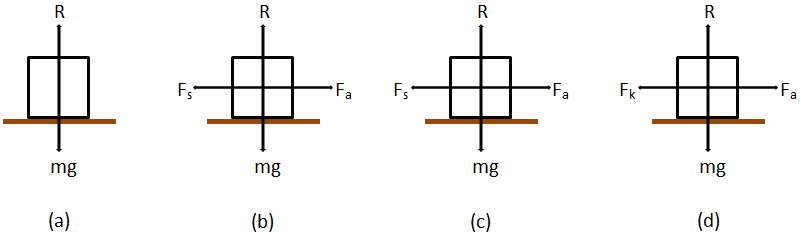Fig. (a) No applied force, block is at rest. (b) Weak applied force, block remains at rest, Fs < μs R. (c) Strong applied force, block just begins to slide. (d) Block sliding at constant speed.

So, the force of friction between two surfaces when the body is in static condition is called static friction and is equal to the applied force. The maximum value of static friction is called limiting friction.

Initially, it requires more force to break the interlocking between the irregularities of two surfaces. But once the block is in motion, some amount of time is required for the interlocking of irregularities to occur. Also, the body gains inertia of motion. So, the force of friction is reduced. The force of friction when the block is sliding is called kinetic or dynamic friction. For a limited speed, the kinetic friction remains constant throughout the motion. Kinetic friction is always less than limiting friction.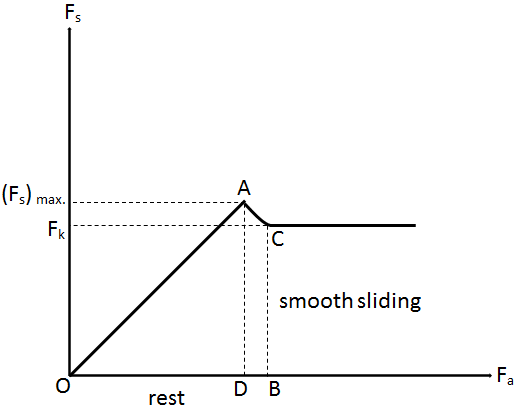Fig. Graph of force of friction and applied force.

A graph between the applied force and force of friction is shown in the figure above. AD represents the maximum static friction called limiting friction. CB represents the kinetic friction. Kinetic friction may be of two types i.e. sliding friction and rolling friction.

### Sliding Friction

The opposing force that comes into play when one body is actually sliding over the surface of another body is called sliding friction. For example, when a flat block is moved over the flat surface of a table, the opposing force experienced by the block is sliding friction.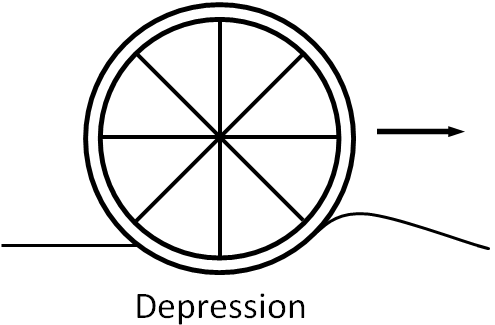### Rolling Friction

The opposing force that comes into play when one body is actually rolling over the surface of the other body is called rolling friction. For example, when a wheel rolls over a surface, the force that opposes it is the rolling friction.

## Co-efficient of Kinetic Friction

Let a body be at rest on a horizontal table as shown in the figure below. When a force Fa is applied on it horizontally, the body just begins to slide along the surface. From the laws of friction, the force of friction F is proportional to the normal reaction R.

###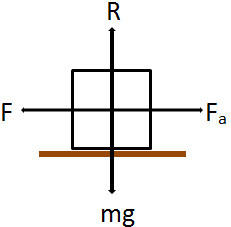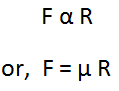where μ is a constant of proportionality called the coefficient of friction between two surfaces in contact.

###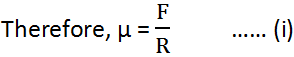The coefficient of friction is the ratio of frictional force to the normal reaction. It is a unit less quantity and its value depends on the material of the objects. If the object is in motion, then we replace F by Fk and μ by μk. So, equation (i) becomes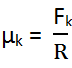where Fk is force of kinetic friction and μk is coefficient of kinetic friction. It is found that μ > μk for a pair of surfaces.

### Coefficient of Friction of Common Materials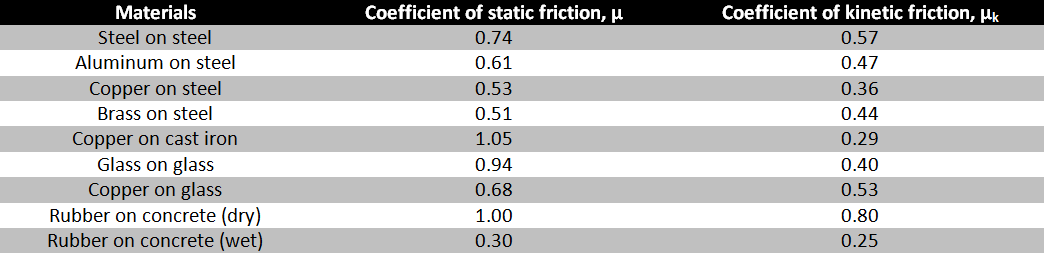Static Friction is a Self Adjusting Force

When a block is at rest and no force is applied, the force of friction is zero. When the magnitude of the applied force is increased till it does not move, the magnitude of the static friction also increases and the direction of static friction and applied force are opposite. That means, the magnitude and direction of static friction adjusts itself according to the magnitude and direction of the applied force.